A positively charged ball hangs from a silk thread. We put a positive test charge q0 at a point and measure F/q0, then it can be predicted that the electric field strength E

(1) > F/q0

(2) = F/q0

(3) < F/q0

(4) Cannot be estimated

Concept Questions :-

Electric field
High Yielding Test Series + Question Bank - NEET 2020

Difficulty Level:

A solid metallic sphere has a charge +3Q. Concentric with this sphere is a conducting spherical shell having charge –Q. The radius of the sphere is a and that of the spherical shell is (b > a). What is the electric field at a distance R(a < R < b) from the centre

(1) $\frac{Q}{2\pi {\epsilon }_{0}R}$

(2) $\frac{3Q}{2\pi {\epsilon }_{0}R}$

(3) $\frac{3Q}{4\pi {\epsilon }_{0}{R}^{2}}$

(4) $\frac{4Q}{4\pi {\epsilon }_{0}{R}^{2}}$

Concept Questions :-

Electric field
High Yielding Test Series + Question Bank - NEET 2020

Difficulty Level:

A point charge q is placed at a distance a/2 directly above the centre of a square of side a. The electric flux through the square is

(1) $\frac{q}{{\epsilon }_{0}}$

(2) $\frac{q}{\pi {\epsilon }_{0}}$

(3) $\frac{q}{4{\epsilon }_{0}}$

(4) $\frac{q}{6{\epsilon }_{0}}$

Concept Questions :-

Gauss law
High Yielding Test Series + Question Bank - NEET 2020

Difficulty Level:

Two infinitely long parallel wires having linear charge densities λ1 and λ2 respectively are placed at a distance of R meters. The force per unit length on either wire will be $\left(K=\frac{1}{4\pi {\epsilon }_{0}}\right)$

(1) $K\frac{2{\lambda }_{1}{\lambda }_{2}}{{R}^{2}}$

(2) $K\frac{2{\lambda }_{1}{\lambda }_{2}}{R}$

(3) $K\frac{{\lambda }_{1}{\lambda }_{2}}{{R}^{2}}$

(4) $K\frac{{\lambda }_{1}{\lambda }_{2}}{R}$

Concept Questions :-

Electric field
High Yielding Test Series + Question Bank - NEET 2020

Difficulty Level:

The charge on 500 cc of water due to protons will be

(1) 6.0 × 1027 C

(2) 2.67 × 107 C

(3) 6 × 1023 C

(4) 1.67 × 1023 C

1. If both the assertion and the reason are true and the reason is a correct explanation of the assertion
2. If both the assertion and reason are true but the reason is not a correct explanation of the assertion
3. If the assertion is true but the reason is false
4. If both the assertion and reason are false

Concept Questions :-

Coulomb's law
High Yielding Test Series + Question Bank - NEET 2020

Difficulty Level:

The electric field in a region is radially outward with magnitude $E=A{\gamma }_{0}$. The charge contained in a sphere of radius ${\gamma }_{0}$ centered at the origin is

(1) $\frac{1}{4\pi {\epsilon }_{0}}A{\gamma }_{0}^{3}$

(2) $4\pi {\epsilon }_{0}A{\gamma }_{0}^{3}$

(3) $\frac{4\pi {\epsilon }_{0}A}{{\gamma }_{0}}$

(4) $\frac{1}{4\pi {\epsilon }_{0}}\frac{A}{{\gamma }_{0}^{3}}$

Concept Questions :-

Gauss law
High Yielding Test Series + Question Bank - NEET 2020

Difficulty Level:

Charge q is uniformly distributed over a thin half-ring of radius R. The electric field at the centre of the ring is

(1) $\frac{q}{2{\pi }^{2}{\epsilon }_{0}{R}^{2}}$

(2) $\frac{q}{4{\pi }^{2}{\epsilon }_{0}{R}^{2}}$

(3) $\frac{q}{4\pi {\epsilon }_{0}{R}^{2}}$

(4) $\frac{q}{2\pi {\epsilon }_{0}{R}^{2}}$

Concept Questions :-

Electric field
High Yielding Test Series + Question Bank - NEET 2020

Difficulty Level:

In the given figure two tiny conducting balls of identical mass m and identical charge q hang from non-conducting threads of equal length L. Assume that θ is so small that $\mathrm{tan}\theta \approx \mathrm{sin}\theta$, then for equilibrium x is equal to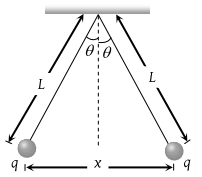(1) ${\left(\frac{{q}^{2}L}{2\pi {\epsilon }_{0}mg}\right)}^{\frac{1}{3}}$

(2) ${\left(\frac{q{L}^{2}}{2\pi {\epsilon }_{0}mg}\right)}^{\frac{1}{3}}$

(3) ${\left(\frac{{q}^{2}{L}^{2}}{4\pi {\epsilon }_{0}mg}\right)}^{\frac{1}{3}}$

(4) ${\left(\frac{{q}^{2}L}{4\pi {\epsilon }_{0}mg}\right)}^{\frac{1}{3}}$

Concept Questions :-

Coulomb's law
High Yielding Test Series + Question Bank - NEET 2020

Difficulty Level:

Three positive charges of equal value q are placed at the vertices of an equilateral triangle. The resulting lines of force should be sketched as in

(1)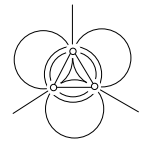(2)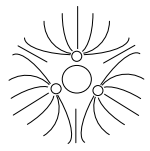(3)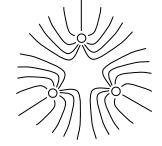(4)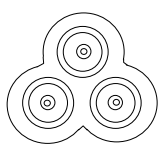Concept Questions :-

Electric field
High Yielding Test Series + Question Bank - NEET 2020

Difficulty Level:

Two equal charges are separated by a distance d. A third charge placed on a perpendicular bisector at x distance will experience maximum coulomb force when

(1) $x=\frac{d}{\sqrt{2}}$

(2) $x=\frac{d}{2}$

(3) $x=\frac{d}{2\sqrt{2}}$

(4) $x=\frac{d}{2\sqrt{3}}$

Concept Questions :-

Coulomb's law